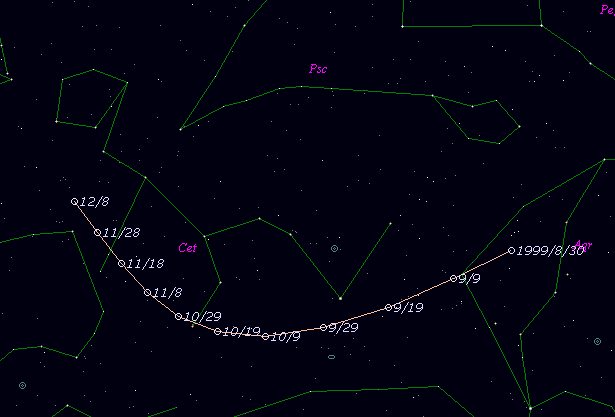# \$B%m%K%*%9WB@1(B

P/1999 RO28 ( LONEOS )###\$B%W%m%U%#!<%k(B

 \$BH/8+F|(B 1999\$BG/(B 9\$B7n(B 7\$BF|(B \$BH/8+8wEY(B 18.4\$BEy(B \$BH/8+ Lowell Observatory Near-Earth Object Search

###\$B50F;MWAG(B

```   The following improved orbital elements by Kenji Muraoka, are
from 109 observations  1999 Sept. 7 to Nov. 13, perturbations
by 9 Planets, Moon and 5 minor planets were taken into account.
The mean residual is +/- 0.73 arc seconds.

Epoch  =  1999 Sept. 19.0  TT      JDT = 2451440.5
T  =  1999 Oct.   2.34327      +/- 0.00033 (m.e.) TT
Peri. =  219.85872                +/- 0.00111
Node  =  148.45030                +/- 0.00093   (2000.0)
Incl. =    8.19110                +/- 0.00017
q  =    1.2317608              +/- 0.0001424 AU
e  =    0.6507922              +/- 0.0000274
a  =    3.5273002              +/- 0.0002999 AU
n  =    0.14877859             +/- 0.00001897
P  =    6.625                  +/- 0.0008448  years
(+/- 0.31 day)
```

###\$B@1?^(B###\$B8wEYJQ2=(B

```        m1 = 20.5 + 5 log\$B&\$(B + 10.0 log r
```##### \$B50F;MWAG\$OB<2,7r<#;a\$N7W;;\$K\$h\$k\$b\$N\$G\$9!#(B \$B@1?^\$O(B StellaNavigator Ver.2.0 for Windows (\$B%"%9%H%m%"!<%D(B \$BJTCx(B / \$B%"%9%-!<=PHG6I4)(B) \$B\$G:n@.\$7\$?\$b\$N\$G\$9!#(B \$B8wEY%0%i%U\$O(BComet for Windows\$B\$G:n@.\$7\$?\$b\$N\$G\$9!#(B